Qucs-core  0.0.19
bondwire Class Reference

#include <bondwire.h>

Inheritance diagram for bondwire: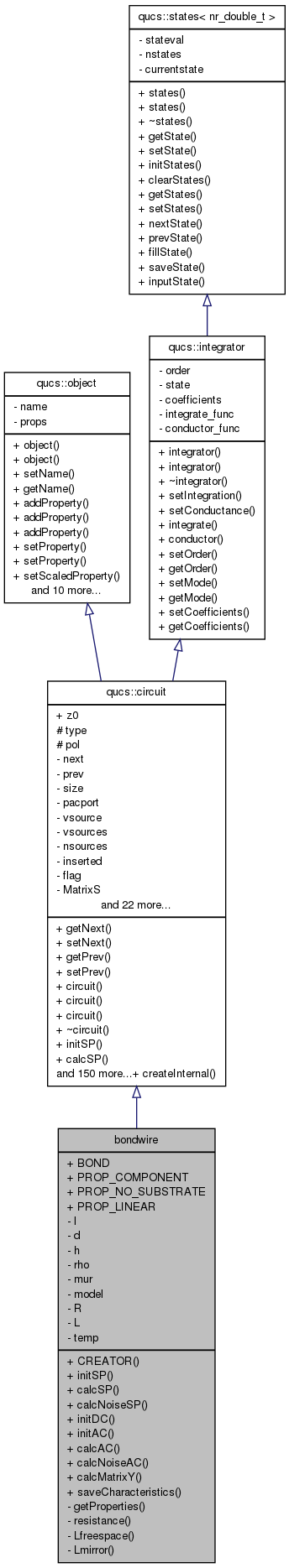[legend]
Collaboration diagram for bondwire: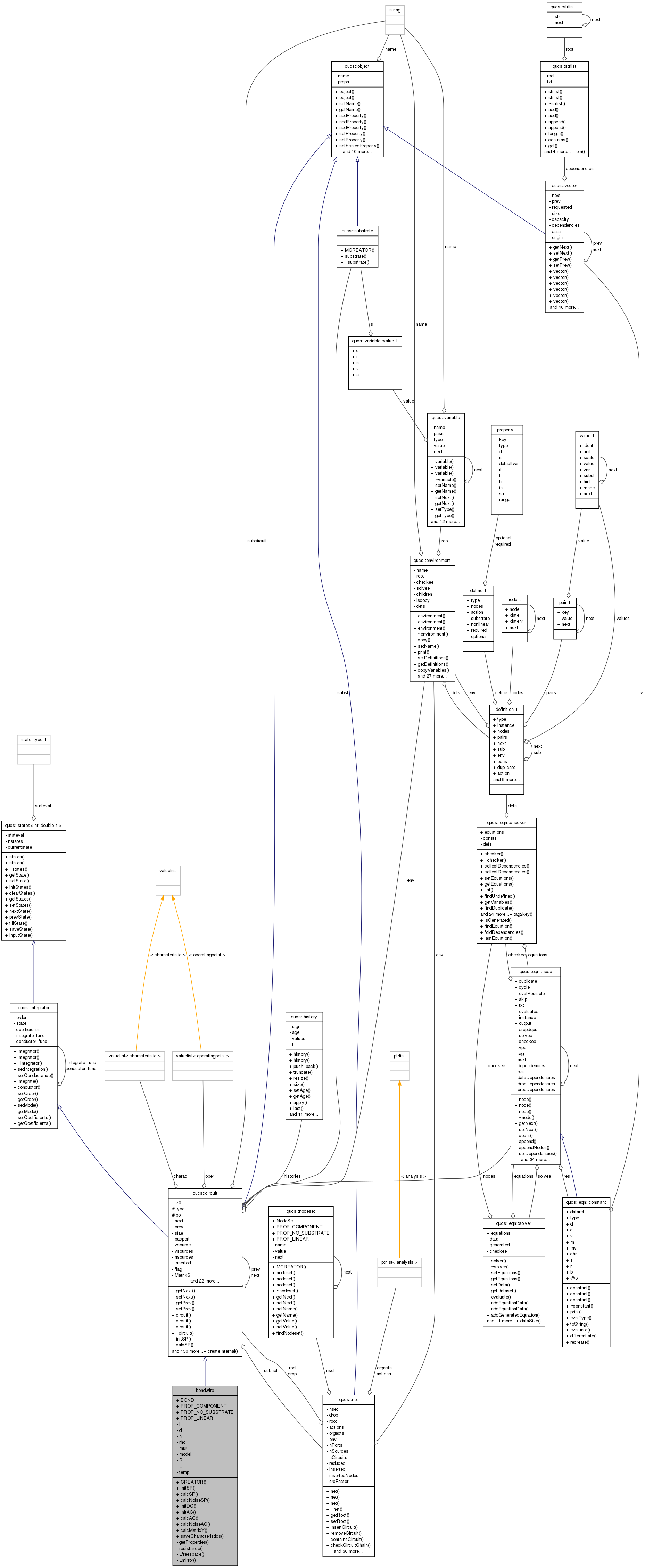[legend]

## Public Member Functions

CREATOR (bondwire)
void initSP (void)
void calcSP (const nr_double_t)
void calcNoiseSP (nr_double_t)
void initDC (void)
void initAC (void)
void calcAC (nr_double_t)
void calcNoiseAC (nr_double_t)
qucs::matrix calcMatrixY (nr_double_t)
void saveCharacteristics (nr_double_t)

## Data Fields

BOND
PROP_COMPONENT
PROP_NO_SUBSTRATE
PROP_LINEAR

## Private Member Functions

void getProperties (void)
Get properties from model. Get properties and fill the class.
nr_double_t resistance (const nr_double_t f) const
nr_double_t Lfreespace (const nr_double_t f) const
nr_double_t Lmirror () const

nr_double_t l
nr_double_t d
nr_double_t h
nr_double_t rho
nr_double_t mur
int model
nr_double_t R
nr_double_t L
nr_double_t temp

## Detailed Description

Definition at line 434 of file bondwire.cpp.

## Member Function Documentation

 void bondwire::calcAC ( nr_double_t frequency )  [virtual]

Compute AC model. ! Use serial LR model (Y matrix).

Reimplemented from qucs::circuit.

Definition at line 399 of file bondwire.cpp.

 matrix bondwire::calcMatrixY ( nr_double_t f )

Compute Y matrix of bond wire.

Definition at line 314 of file bondwire.cpp.

 void bondwire::calcNoiseAC ( nr_double_t )  [virtual]

Reimplemented from qucs::circuit.

Definition at line 411 of file bondwire.cpp.

 void bondwire::calcNoiseSP ( nr_double_t )  [virtual]

Reimplemented from qucs::circuit.

Definition at line 403 of file bondwire.cpp.

 void bondwire::calcSP ( const nr_double_t frequency )  [virtual]

Compute S parameters. ! Reuse computed Y matrix.

Reimplemented from qucs::circuit.

Definition at line 353 of file bondwire.cpp.

 bondwire::CREATOR ( bondwire )
 void bondwire::getProperties ( void )  [private]

Get properties from model. Get properties and fill the class.

Todo:
check values

Definition at line 94 of file bondwire.cpp.

 void bondwire::initAC ( void )  [virtual]

Initialize AC simulation.

Reimplemented from qucs::circuit.

Definition at line 390 of file bondwire.cpp.

 void bondwire::initDC ( void )  [virtual]

DC model initialization. ! DC model of a bondwire is a resistance.

Reimplemented from qucs::circuit.

Definition at line 366 of file bondwire.cpp.

 void bondwire::initSP ( void )  [virtual]

Initialize S parameter simulation.

Reimplemented from qucs::circuit.

Definition at line 345 of file bondwire.cpp.

 nr_double_t bondwire::Lfreespace ( const nr_double_t f ) const [private]

Compute free space inductance. According to  pp63 (2.29) free space inductance (in nH) is such as (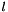in micrometers):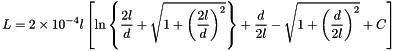According to  self inductance is (in H with l in m):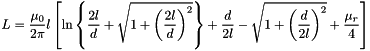Finally we will use (in H with l in m):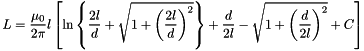Parameters:
 f frequency d bond wire diameter l bond wire length (in meters) rho bond wire resistivity mur relative magnetic permeabillity

Definition at line 254 of file bondwire.cpp.

 nr_double_t bondwire::Lmirror ( void ) const [private]

Compute inductance modeling ground plane effect. According to  pp64 (2.32) inductance (in nH) is such as (in micrometers):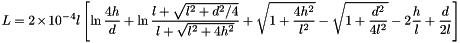Finally we will use (in H with l in m):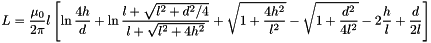Note:
Mirror is a strange model that is frequency independent. Whereas computations are valid, hypothesis are arguable. Indeed, they did the assumption that the ground plane is perfect that is really a zero order model in the high frequency domain.

Definition at line 297 of file bondwire.cpp.

 nr_double_t bondwire::resistance ( const nr_double_t f ) const [private]

Compute resitance of the wire. Resitance of the wire is computed using classical tube model enhanced for case where tube is greater than conductor (i.e. low frequency case).

Todo:
Offer other resistance model for instance exponential decay and bessel function exact computation. But I do not know it is worth the effort.
Todo:
Factorise the resistance model.

Definition at line 159 of file bondwire.cpp.

 void bondwire::saveCharacteristics ( nr_double_t )  [virtual]

Save self-inductance and resistance.

Reimplemented from qucs::circuit.

Definition at line 358 of file bondwire.cpp.

## Field Documentation

Definition at line 435 of file bondwire.cpp.

 nr_double_t bondwire::d [private]

diameter of bond wire (m)

Definition at line 51 of file bondwire.h.

 nr_double_t bondwire::h [private]

height from ground plane only used in mirror model

Definition at line 52 of file bondwire.h.

 nr_double_t bondwire::l [private]

length of bond wire (m)

Definition at line 50 of file bondwire.h.

 nr_double_t bondwire::L [private]

Definition at line 56 of file bondwire.h.

 int bondwire::model [private]

model number

Definition at line 55 of file bondwire.h.

 nr_double_t bondwire::mur [private]

relative magnetic permeabilty

Definition at line 54 of file bondwire.h.

Definition at line 435 of file bondwire.cpp.

Definition at line 435 of file bondwire.cpp.

Definition at line 435 of file bondwire.cpp.

 nr_double_t bondwire::R [private]

Definition at line 56 of file bondwire.h.

 nr_double_t bondwire::rho [private]

resistivity

Definition at line 53 of file bondwire.h.

 nr_double_t bondwire::temp [private]

ambient temperature

Definition at line 57 of file bondwire.h.

The documentation for this class was generated from the following files: